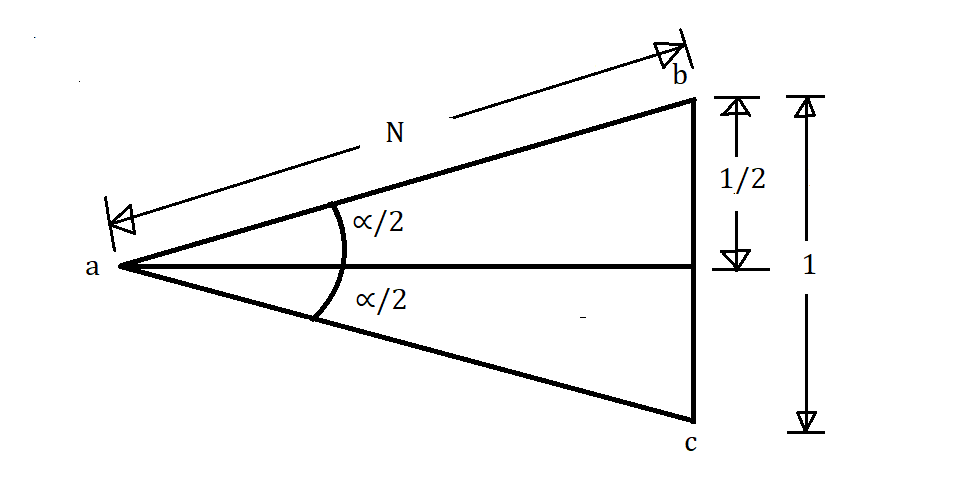# Angle of Crossing in Railway

16th January 2020

## The angle of Crossing in Railway

The angle which is formed between the gauge faces of the Vee is known as the crossing angle or angle of the railway crossing. Following are the three methods used to determine the angle of crossing in railway.

### 1. Right Angle or Cole’s Method

In this method, a right angle triangle is used to determine the angle of crossing.

Notation:

•$\alpha$= Angle of Crossing
• N = Crossing Number

From this Figure, we can write$\tan \alpha = \frac{1}{N}$

Or,$\cot \alpha = N$ …………(1.a)

Or,$N = \cot \alpha$ …………(1.b)

Equation (1.a) shows the angle of crossing.
Equation (1.b) shows the number of crossing.

Note: This is the standard method mostly adopted on the Indian Railways.

### 2. Centre Line Method

In this method, the measurement is taken alon a line bisecting the crossing angle as shown in fig.

Now,$\tan \frac{\alpha}{2} = \frac{\frac{1}{2}}{N} = \frac{1}{2N}$

Or,$\cot \frac{\alpha}{2} = 2N$ …………..(2.a)

Or,$N = \frac{1}{2}.\cot \frac{\alpha}{2}$ ……….(2.b)

Equation (2.a) shows the angle of crossing.
Equation (2.b) shows the number of crossing.

Note: This method is adopted in some countries like U.K. and U.S.A.

### 3. Isosceles Triangle Method

In this case, the measurement is taken along one side of the isosceles triangle as shown in fig.

Then,$\sin \frac{\alpha}{2} = \frac{\frac{1}{2}}{N} = \frac{1}{2N}$

Or,$cosec \frac{\alpha}{2} = 2N$ …………..(3.a)

Or,$N = \frac{1}{2}. cosec \frac{\alpha}{2}$ ……….(3.b)

Equation (3.a) shows the angle of crossing.
Equation (3.b) shows the number of crossing.

Note: This method is implemented in the case of tramway layout.

More the angle of crossing lesser will be permissible speed and vice versa